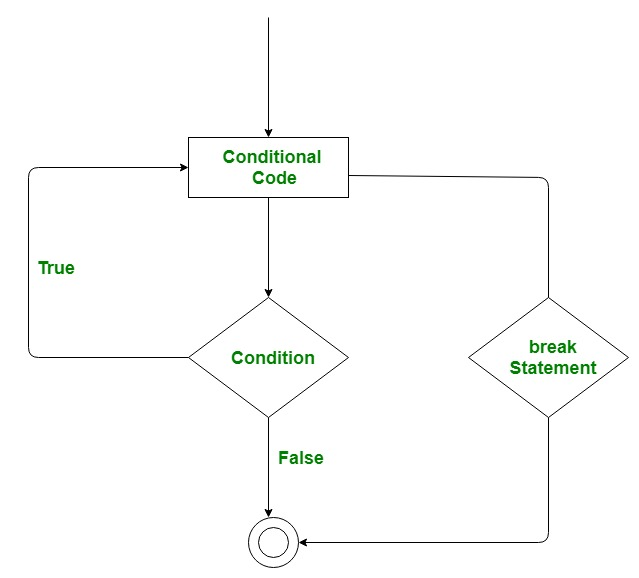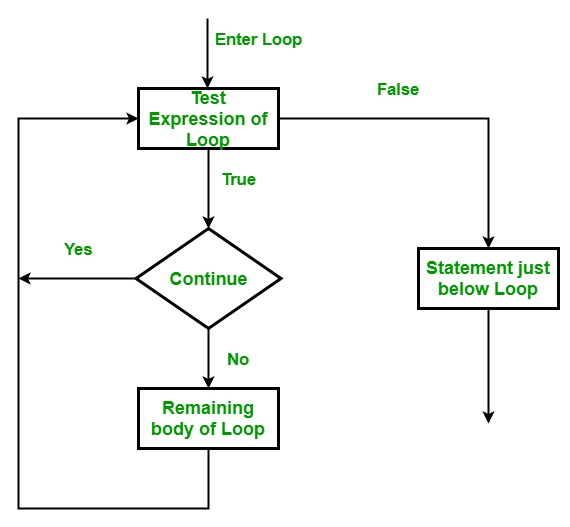Related Articles

# break, continue and pass in Python

• Difficulty Level : Hard
• Last Updated : 25 Nov, 2019

Using loops in Python automates and repeats the tasks in an efficient manner. But sometimes, there may arise a condition where you want to exit the loop completely, skip an iteration or ignore that condition. These can be done by loop control statements. Loop control statements change execution from its normal sequence. When execution leaves a scope, all automatic objects that were created in that scope are destroyed. Python supports the following control statements.

## Break statement

The `break `statement is used to terminate the loop or statement in which it is present. After that, the control will pass to the statements that are present after the break statement, if available. If the break statement is present in the nested loop, then it terminates only those loops which contains `break `statement.

Syntax:

```break
```Example:
Consider a situation where you want to iterate over a string and want to print all the characters until a letter ‘e’ or ‘s’ is encountered. It is specified that you have to do this using loop and only one loop is allowed to use.
Here comes the usage of `break `statement. What we can do is iterate over a string using either a `while `loop or `for `loop and every time we have to compare the value of iterator with ‘e’ or ‘s’. If it is ‘e’ or ‘s’ we will use the break statement to exit the loop.

Below is the implementation.

 `# Python program to demonstrate``# break statement`` ` `# Python program to ``# demonstrate break statement ``   ` `s ``=` `'geeksforgeeks'``# Using for loop ``for` `letter ``in` `s: ``   ` `    ``print``(letter) ``    ``# break the loop as soon it sees 'e' ``    ``# or 's' ``    ``if` `letter ``=``=` `'e'` `or` `letter ``=``=` `'s'``: ``        ``break``   ` `print``(``"Out of for loop"``) ``print``() ``   ` `i ``=` `0``   ` `# Using while loop ``while` `True``: ``    ``print``(s[i]) ``   ` `    ``# break the loop as soon it sees 'e' ``    ``# or 's' ``    ``if` `s[i] ``=``=` `'e'` `or` `s[i] ``=``=` `'s'``: ``        ``break``    ``i ``+``=` `1``   ` `print``(``"Out of while loop"``)`

Output:

```g
e
Out of for loop

g
e
Out of while loop
```

## Continue statement

`Continue `is also a loop control statement just like the break statement. `continue `statement is opposite to that of break statement, instead of terminating the loop, it forces to execute the next iteration of the loop.
As the name suggests the continue statement forces the loop to continue or execute the next iteration. When the continue statement is executed in the loop, the code inside the loop following the continue statement will be skipped and the next iteration of the loop will begin.

Syntax:

```continue
```Example:
Consider the situation when you need to write a program which prints the number from 1 to 10 and but not 6. It is specified that you have to do this using loop and only one loop is allowed to use.
Here comes the usage of `continue `statement. What we can do here is we can run a loop from 1 to 10 and every time we have to compare the value of iterator with 6. If it is equal to 6 we will use the continue statement to continue to next iteration without printing anything otherwise we will print the value.

Below is the implementation of the above idea:

 `# Python program to ``# demonstrate continue ``# statement ``   ` `# loop from 1 to 10 ``for` `i ``in` `range``(``1``, ``11``): ``   ` `    ``# If i is equals to 6,   ``    ``# continue to next iteration   ``    ``# without printing  ``    ``if` `i ``=``=` `6``: ``        ``continue``    ``else``: ``        ``# otherwise print the value ``        ``# of i ``        ``print``(i, end ``=` `" "``)`

Output:

```1 2 3 4 5 7 8 9 10
```

## Pass statement

As the name suggests pass statement simply does nothing. The pass statement in Python is used when a statement is required syntactically but you do not want any command or code to execute. It is like `null `operation, as nothing will happen is it is executed. `Pass `statement can also be used for writing empty loops. Pass is also used for empty control statement, function and classes.

Syntax:

```pass
```

Example:

 `# Python program to demonstrate``# pass statement`` ` ` ` `s ``=` `"geeks"`` ` `# Empty loop``for` `i ``in` `s:``    ``# No error will be raised``    ``pass`` ` `# Empty function``def` `fun():``    ``pass`` ` `# No error will be raised``fun()`` ` `# Pass statement``for` `i ``in` `s:``    ``if` `i ``=``=` `'k'``:``        ``print``(``'Pass executed'``)``        ``pass``    ``print``(i)`

Output:

```g
e
e
Pass executed
k
s
```

In the above example, when the value of i becomes equal to ‘k’, the pass statement did nothing and hence the letter ‘k’ is also printed.

Attention geek! Strengthen your foundations with the Python Programming Foundation Course and learn the basics.

To begin with, your interview preparations Enhance your Data Structures concepts with the Python DS Course. And to begin with your Machine Learning Journey, join the Machine Learning – Basic Level Course

My Personal Notes arrow_drop_up